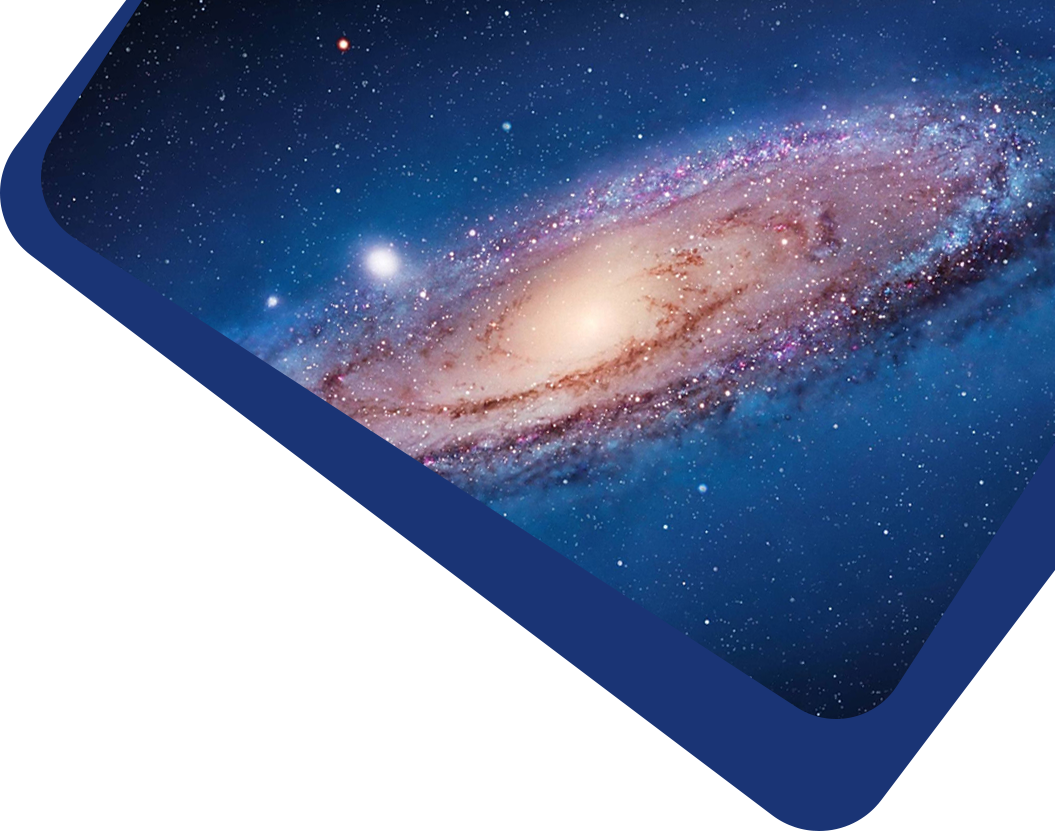Vol 21, No 2

## A semi-analytic model for the study of 1/1 resonant dynamics of the planar elliptic restricted co-orbital problem

#### Miao Li, Yu-Kun Huang, Sheng-Ping Gong

Abstract

Abstract Mean motion resonances (MMRs) are widespread in our Solar System. Moreover, resonant dynamics has always been an essential topic in planetary research. Recently, the research about exoplanets and the potential Planet Nine with large eccentricity has given rise to our interests in the secular dynamics inside MMRs in the elliptic model. In this paper, we study the fixed points of the averaged Hamiltonian and the long-term stable regions of the 1/1 resonance (or co-orbital motion) in the elliptic restricted three-body problem (ERTBP) systematically. Numerical integrations prove those test particles trapped in “apsidal co-rotation”, where both the resonant angle $$\phi_{\rm res}$$ and the secular angle $$\Delta\varpi$$ (or apsidal longitude differences) librate simultaneously, always survive the long-term simulations. Furthermore, utilizing a semianalytical method based on the adiabatic approach, three families of long-term fixed points of the averaged Hamiltonian of the planar ERTBP inside the 1/1 resonance have been found. We call them QS-points, H-points, and T-points here, whose values of the ($$\phi_{\rm res},\Delta\varpi$$) are (0°, 180°), (180°, 0°), and (±60°, ±60°), respectively. All the fixed points of the averaged Hamiltonian of the co-orbital motion in the ERTBP are presented in the $$e-e’$$ plane (' represents the elements of the planet in this paper). We find that QS-points and T-points always exist for the arbitrary eccentricity of a planet, while H-points only exist for the cases of low $$e’$$ and very high $$e$$. Furthermore, we measure the libration width in terms of eccentricity, $$\Delta e$$, around these stable equilibrium points in the $$e-\Delta\varpi$$ phase-space portraits. The “apsidal co-rotation” around all the stable equilibrium points is presented in the $$e-e’$$ plane. All these results are effectively confirmed by numerical experiments. The long-term stable zones around these periodic orbits in the $$e-e’$$ plane are significant for the research of the co-orbital motion in the ERTBP. Above all, these practical approaches that we proposed can also be used to study the secular dynamics of other MMRs.

Keywords

Keywords celestial mechanics — minor planets, asteroids — planets and satellites: dynamical evolution and stability

Full Text
Refbacks

• There are currently no refbacks.